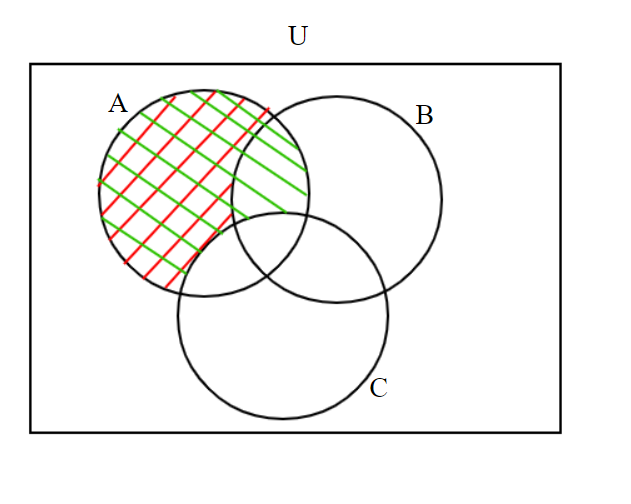Courses
Courses for Kids
Free study material
Free LIVE classes
MoreLIVE
Join Vedantu’s FREE Mastercalss

# If A, B and C are sets, then prove that $\left( {{\text{A}} - {\text{B}}} \right) \cap \left( {{\text{A}} - {\text{C}}} \right) = {\text{A}} - \left( {{\text{B}} \cup {\text{C}}} \right)$. Verify the above result by Venn diagrams.Verified
361.5k+ views
Hint: Here, we will proceed to convert the LHS into the RHS of the equation we needed to prove using the formula for difference of sets, associative property of sets and De Morgan’s law.

Let A, B and C be three sets whose Venn diagram is shown in the figure. U is the universal set.
To prove- $\left( {{\text{A}} - {\text{B}}} \right) \cap \left( {{\text{A}} - {\text{C}}} \right) = {\text{A}} - \left( {{\text{B}} \cup {\text{C}}} \right)$
Clearly according to the definition of difference of sets, we can write
$\left( {{\text{A}} - {\text{B}}} \right) = {\text{A}} \cap {{\text{B}}^{\text{c}}}{\text{ }} \to {\text{(1)}}$ where ${{\text{B}}^{\text{c}}}$ is the complement of set B
$\left( {{\text{A}} - {\text{C}}} \right) = {\text{A}} \cap {{\text{C}}^{\text{c}}}{\text{ }} \to {\text{(2)}}$ where ${{\text{C}}^{\text{c}}}$ is the complement of set C
Taking LHS of the equation we need to prove and then using equations (1) and (2), we get
$\left( {{\text{A}} - {\text{B}}} \right) \cap \left( {{\text{A}} - {\text{C}}} \right) = \left( {{\text{A}} \cap {{\text{B}}^{\text{c}}}} \right) \cap \left( {{\text{A}} \cap {{\text{C}}^{\text{c}}}} \right){\text{ }} \to {\text{(3)}}$
According to associative property of the sets, we can write
For any four sets A, B, C and D $\left( {{\text{A}} \cap {\text{B}}} \right) \cap \left( {{\text{C}} \cap {\text{D}}} \right) = \left( {{\text{A}} \cap {\text{C}}} \right) \cap \left( {{\text{B}} \cap {\text{D}}} \right)$
Replacing set B with set BC, set C with set A and set D with set CC in the above property, RHS of equation (3) becomes
$\Rightarrow \left( {{\text{A}} - {\text{B}}} \right) \cap \left( {{\text{A}} - {\text{C}}} \right) = \left( {{\text{A}} \cap {\text{A}}} \right) \cap \left( {{{\text{B}}^{\text{c}}} \cap {{\text{C}}^{\text{c}}}} \right){\text{ }} \to {\text{(4)}}$
As we know that the intersection of any set A with the same set A will result in set A only i.e., $\left( {{\text{A}} \cap {\text{A}}} \right) = {\text{A }} \to {\text{(5)}}$
Using equation (5), the RHS of equation (4) becomes
$\Rightarrow \left( {{\text{A}} - {\text{B}}} \right) \cap \left( {{\text{A}} - {\text{C}}} \right) = {\text{A}} \cap \left( {{{\text{B}}^{\text{c}}} \cap {{\text{C}}^{\text{c}}}} \right)$
According to De Morgan’s law for any two sets B and C, $\left( {{{\text{B}}^{\text{c}}} \cap {{\text{C}}^{\text{c}}}} \right) = {\left( {{\text{B}} \cup {\text{C}}} \right)^{\text{c}}}$
$\Rightarrow \left( {{\text{A}} - {\text{B}}} \right) \cap \left( {{\text{A}} - {\text{C}}} \right) = {\text{A}} \cap {\left( {{\text{B}} \cup {\text{C}}} \right)^{\text{c}}}{\text{ }} \to {\text{(6)}}$
According to definition of difference of sets
For any two sets E and F, ${\text{E}} \cap {{\text{F}}^{\text{c}}} = {\text{E}} - {\text{F}}$
By replacing set E by set A and set F by set $\left( {{\text{B}} \cup {\text{C}}} \right)$, the RHS of equation (6) becomes
$\Rightarrow \left( {{\text{A}} - {\text{B}}} \right) \cap \left( {{\text{A}} - {\text{C}}} \right) = {\text{A}} - \left( {{\text{B}} \cup {\text{C}}} \right)$
The above equation is the equation we needed to prove.
For verification of $\left( {{\text{A}} - {\text{B}}} \right) \cap \left( {{\text{A}} - {\text{C}}} \right) = {\text{A}} - \left( {{\text{B}} \cup {\text{C}}} \right)$ using Venn diagram, we have
$\left( {{\text{A}} - {\text{B}}} \right)$ is represented by red lines and $\left( {{\text{A}} - {\text{C}}} \right)$ is represented by green lines. Then, $\left( {{\text{A}} - {\text{B}}} \right) \cap \left( {{\text{A}} - {\text{C}}} \right)$ means the region which is common to both $\left( {{\text{A}} - {\text{B}}} \right)$ and $\left( {{\text{A}} - {\text{C}}} \right)$ i.e., the region where both red lines and red lines exists. Clearly, the region where both red and green lines are present is ${\text{A}} - \left( {{\text{B}} \cup {\text{C}}} \right)$.
So, it is verified using Venn diagram that $\left( {{\text{A}} - {\text{B}}} \right) \cap \left( {{\text{A}} - {\text{C}}} \right) = {\text{A}} - \left( {{\text{B}} \cup {\text{C}}} \right)$.Note: In these types of problems, complement of any set means the remaining region in the whole universal set left after removing that set whose complement is required like the complement set of B i.e., Bc represents the region left in the complete universal set when set B is removed from it.
Last updated date: 25th Sep 2023
Total views: 361.5k
Views today: 5.61k